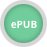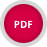John Wiley & Sons Elementary Differential Equations and Boundary Value ProblemsBoyce's Elementary Differential Equations and Boundary Value Problems is written from the viewpoint .. Product #: 978-1-119-82051-2 Regular price: \$61.59 \$61.59 Auf Lager

# Elementary Differential Equations and Boundary Value Problems12. Auflage Juni 2022
736 Seiten, Softcover
Lehrbuch

ISBN: 978-1-119-82051-2
John Wiley & Sons

Jetzt kaufen

### Preis: 65,90 €

Preis inkl. MwSt, zzgl. Versand

Weitere VersionenBoyce's Elementary Differential Equations and Boundary Value Problems is written from the viewpoint of the applied mathematician, with diverse interest in differential equations, ranging from quite theoretical to intensely practical-and usually a combination of both. The intended audience for the text is undergraduate STEM students taking an introductory course in differential equations. The main prerequisite for engaging with the program is a working knowledge of calculus, gained from a normal two or three semester course sequence or its equivalent, while a basic familiarity with matrices is helpful.

This new edition of the book aims to preserve, and to enhance the qualities that have made previous editions so successful. It offers a sound and accurate exposition of the elementary theory of differential equations with considerable material on methods of solution, analysis, and approximation that have proved useful in a wide variety of applications.

1 Introduction

1.1 Some Basic Mathematical Models

1.2 Solutions of Some Differential Equations

1.3 Classification of Differential Equations

2 First-Order Differential Equations

2.1 Linear Differential Equations; Method of Integrating Factors

2.2 Separable Differential Equations

2.3 Modeling with First-Order Linear Differential Equations

2.4 Differences Between Linear and Nonlinear Differential Equations

2.5 Autonomous Differential Equations and Population Dynamics

2.6 Exact Differential Equations and Integrating Factors

2.7 Numerical Approximations: Euler's Method

2.8 The Existence and Uniqueness Theorem

2.9 First-Order Difference Equations

3 Second-Order Linear Differential Equations

3.1 Homogeneous Differential Equations with Constant Coefficients

3.2 Solutions of Linear Homogeneous Equations; the Wronskian

3.3 Complex Roots of the Characteristic Equation

3.4 Repeated Roots; Reduction of Order

3.5 Nonhomogeneous Equations; Method of Undetermined Coefficients

3.6 Variation of Parameters

3.7 Mechanical and Electrical Vibrations

3.8 Forced Periodic Vibrations

3.9 Central Gravitational Forces and Kepler's Laws

4 Higher-Order Linear Differential Equations

4.1 General Theory of nth Order Linear Differential Equations

4.2 Homogeneous Differential Equations with Constant Coefficients

4.3 The Method of Undetermined Coefficients

4.4 The Method of Variation of Parameters

5 Series Solutions of First-Order and Second-Order Linear Equations

5.1 Review of Power Series

5.2 Series Solution of First Order Equations

5.3 Series Solutions Near an Ordinary Point, Part I

5.4 Series Solutions Near an Ordinary Point, Part II

5.5 Euler Equations; Regular Singular Points

5.6 Series Solutions Near a Regular Singular Point, Part I

5.7 Series Solutions Near a Regular Singular Point, Part II

5.8 Bessel's Equation

6 Systems of First-Order Linear Equations

6.1 Introduction

6.2 Matrices

6.3 Systems of Linear Algebraic Equations; Linear Independence, Eigenvalues, Eigenvectors

6.4 Basic Theory of Systems of First-Order Linear Equations

6.5 Homogeneous Linear Systems with Constant Coefficients

6.6 Complex-Valued Eigenvalues

6.7 Fundamental Matrices

6.8 Repeated Eigenvalues

6.9 Nonhomogeneous Linear Systems

7 The Laplace Transform

7.1 Definition of the Laplace Transform

7.2 Solution of Initial Value Problems

7.3 Step Functions

7.4 Differential Equations with Discontinuous Forcing Functions

7.5 Impulse Functions

7.6 The Convolution Integral

8 Numerical Methods of Solving First Order Equations

8.1 The Euler or Tangent Line Method

8.2 Improvements on the Euler Method

8.3 The Runge-Kutta Method

8.4 Multistep Methods

8.5 Systems of First-Order Equations

8.6 More on Errors; Stability

9 Nonlinear Differential Equations and Stability

9.1 The Phase Plane: Linear Systems

9.2 Autonomous Systems and Stability

9.3 Locally Linear Systems

9.4 Competing Species

9.5 Predator - Prey Equations

9.6 Lyapunov's Second Method

9.7 Periodic Solutions and Limit Cycles

9.8 Chaos and Strange Attractors: The Lorenz Equations

10 Partial Differential Equations and Fourier Series

10.1 Two-Point Boundary Value Problems

10.2 Fourier Series

10.3 The Fourier Convergence Theorem

10.4 Even and Odd Functions

10.5 Separation of Variables; Heat Conduction in a Rod

10.6 Other Heat Conduction Problems

10.7 The Wave Equation: Vibrations of an Elastic String

10.8 Laplace's Equation

A APPENDIX 537

B APPENDIX 541

11 Boundary Value Problems and Sturm-Liouville Theory

11.1 The Occurrence of Two-Point Boundary Value Problems

11.2 Sturm-Liouville Boundary Value Problems

11.3 Nonhomogeneous Boundary Value Problems

11.4 Singular Sturm-Liouville Problems

11.5 Further Remarks on the Method of Separation of Variables: A Bessel Series Expansion

11.6 Series of Orthogonal Functions: Mean Convergence

Web Appendix

Special Functions: On Legendre Polynomials and Functions